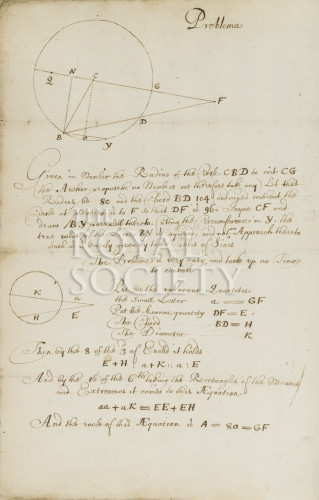Image number: RS.15403

## The Austrian problem

17th century

Unknown, Artist

p2

#### Dimensions

height (page): 328mm
width (page): 211mm

#### Description

Mathematical diagrams in a document 'Concerning Problema Austriacum'. Put forward as a document containing problems and answers, there are two mathematical problems in here, described in Latin and printed by Lucas Straubius in 1653. The answers, given in English and written in the same hand as the questions, show how the length of BY can be calculated when the length of BD is known.

A larger copy, probably for sharing and discussing with a group of people, can be found at Cl.P/24/5a/002-003.

#### Transcription

Given the Number the Radius of the Circle CBD to roit CG the (Author proposeth no Numbers we therefore take any) Let that Radius be 80 and the Chord BD 104 continued without the circle at pleasure as to F so that DF is 96, Joyne CF and draw BY parralell thereto, cutting the circumference in Y, the true value of the Line BY is required, and not an Approache thereto such as easily given by the Tables of Sines. The Problem is very easy, and took up on Time to consider.
Put for the unknowne Quantitie the small letter a = GF
Put the knowne quantity DF = E
The CHord BD = H
The diamter K
Transcribed by the Making Visible project